Courses

# Short Answer Type Questions (Part - 2) - Arithmetic Progressions Class 10 Notes | EduRev

## Class 10 : Short Answer Type Questions (Part - 2) - Arithmetic Progressions Class 10 Notes | EduRev

The document Short Answer Type Questions (Part - 2) - Arithmetic Progressions Class 10 Notes | EduRev is a part of the Class 10 Course Class 10 Mathematics by VP Classes.
All you need of Class 10 at this link: Class 10

Q17. If Sthe sum of n terms of an A.P. is given by Sn = 3n2 - 4n, find the nth term.
Sol. We have:
Sn - 1 = 3 (n - 1)2 - 4 (n - 1)
= 3 (n2 - 2n + 1) - 4n + 4
= 3n2 - 6n + 3 - 4n + 4
= 3n2 - 10n + 7
∵ nth term = Sn - Sn - 1
= 3n2 - 4n - [3n2 - 10n + 7]
= 3n2 - 4n - 3n2 + 10n - 7
= 6n - 7.

Q18. The sum of 4th and 8th terms of an A.P. is 24, and the sum of 6th and 10th terms is 44. Find the A.P.
Sol. Let, the first term = a
Common difference be = d
∴ Using Tn = a + (n - 1) d, we have
T4 = a + 3d
T6 = a + 5d
T8 = a + 7d
T10 = a + 9d
∵ T+ T8 = 24
∴ (a + 3d) + (a + 7d) = 24
⇒ 2a + 10d = 24
⇒ a + 5d = 12
[Dividing by 2]    ...(1)
Also T6 + T10 = 44
∴ (a + 5d) + (a + 9d) = 44
⇒ 2a + 14d = 44
⇒ a + 7d = 22
[Dividing by 2]   ...(2)
Subtracting (1) from (2), we have:
(a + 7d) - (a + 5d) = 22 - 12
⇒ 2d = 10 ⇒ d = 5
From (1), a + 5 (5) = 12
⇒ a = 12 - 25 = - 13
Since, the A.P. is given by:
a, a + d, a + 2d, .....
∴ We have the required A.P. as:
- 13, (- 13 + 5), [- 13 + 2 (5)], .....
or - 13, - 8, - 3, .....

Q19. If Sn, the sum of first n terms of an A.P. is given by
Sn = 5n2 + 3n
Then find the nth term.
Sol.
∵ Sn = 5n2 + 3n
∴ Sn - 1 = 5 (n - 1)2 + 3 (n - 1)
= 5 (n2 - 2n + 1) + 3 (n - 1)
= 5n2 - 10n + 5 + 3n - 3
= 5n2 - 7n + 2
Now, nth term = Sn - Sn - 1
∴ The required nth term
= [5n2 + 3n] - [5n2 - 7n + 2]
= 10n - 2.

Q20. The sum of 5th and 9th terms of an A.P. is 72 and the sum of 7th and 12th term of 97. Find the A.P.
Sol. Let ‘a’ be the 1st term and ‘d’ be the common difference of the A.P.
Now, using Tn = a + (n - 1) d, we have
T5 = a + 4d
T7 = a + 6d
T9 = a + 8d
T12 = a + 11d
∵ T5 + T9 = 72
∴ a + 4d + a + 8d = 72
⇒ 2a + 12d = 72
⇒ a + 6d = 36
[Dividing by 2]   ...(1)
Also T7 + T12= a + 6d + a + 11d = 97
⇒ 36 + a + 11d = 97 [From (1)]
⇒ a + 11d = 97 - 36
⇒ a + 11d = 61    ...(2)
Subtracting (1) from (2), we get
a + 11d - a - 6d = 61 - 36
⇒ 5d = 25
⇒ d = 25/5
From (1), we have
a + 11 (5) = 61
a + 55 = 61
⇒ a = 61 - 55 = 6
Now, an A.P. is given by
a, a + d, a + 2d, a + 3d, .....
∴ The required A.P. is:
6, (6 + 5), [6 + 2 (5)], [6 + 3 (5)], .....
or 6, 11, 16, 24, .....

Q21. In an A.P. the sum of its first ten terms is –150 and the sum of its next term is –550. Find the A.P.
Sol. Let the first term = a
And the common difference = d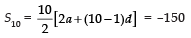⇒ 10a + 45d = –150
⇒ 2a + 9d = –30   ...(1)
∵ The sum of next 10 terms
(i.e. S20 – S10) = –550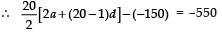⇒ 20a + 190d + 150 = –550
⇒ 2a + 19d + 15 = –55
⇒ 2a + 19d = – 55 – 15
⇒ 2a + 19d = –70   ...(2)
Subtracting (1) from (2), we get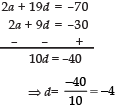From (1), 2(a) + 9(–4) = –30 or a = 6/2 = 3
Thus, AP is a, a + d, a + 2d ...
or 3, [3 + (–4)], [3 + 2(–4)], ...
or 3, –1, –5, ...

Q22. Which term of the A.P. 3, 15, 27, 39, ..... will be 120 more than its 21st term?
Sol. Let the 1st term is ‘a’ and common difference = d
∴ a = 3 and d = 15 - 3 = 12
Now, using Tn = a + (n - 1) d
∴ T21 = 3 + (21 - 1) × 12
= 3 + 20 × 12
= 3 + 240 = 243
Let the required term be the nth term.
∵ nth term = 120 + 21st term
= 120 + 243 = 363
Now Tn = a + (n - 1) d
⇒ 363 = 3 + (n - 1) × 12
⇒ 363 - 3 = (n - 1) × 12
⇒ n - 1 = 360/12 = 30
⇒ n = 30 + 1 = 31
Thus the required term is the 31st term of the A.P.

Q23. Which term of the A.P. 4, 12, 20, 28, ..... will be 120 more than its 21st term?
Sol. Here, a = 4
d = 12 - 4 = 8
Using Tn = a + (n - 1) d
∴ T21 = 4 + (21 - 1) × 8
= 4 + 20 × 8 = 164
∵ The required nth term = T21 + 120
∴ nth term = 164 + 120 = 284
∴ 284 = a + (n - 1) d
⇒ 284 = 4 + (n - 1) × 8
⇒ 284 - 4 = (n - 1) × 8
⇒ n - 1 = 280/8 = 35
⇒ n = 35 + 1 = 36
Thus, the required term is the 36th term of the A.P.

Q24. The sum of n terms of an A.P. is 5n2 - 3n. Find the A.P. Hence find its 10th term.
Sol. We have:
Sn = 5n2 - 3n
∴ S1 = 5 (1)2 - 3 (1) = 2
⇒ First term T1 = (a) = 2
S2 = 5 (2)2 - 3 (2)
= 20 - 6 = 14
⇒ Second term T2 = 14 - 2 = 12
Now the common difference = T2 - T1
⇒ d = 12 - 2 = 10
∵ An A.P. is given by
a, (a + d), (a + 2d) .....
∴ The required A.P. is:
2, (2 + 10), [2 + 2 (10)], .....
⇒ 2, 12, 22, .....
Now, using Tn = a + (n - 1) d, we have
T10 = 2 + (10 - 1) × 10
= 2 + 9 × 10
= 2 + 90 = 92.

Q25. Find the 10th term from the end of the A.P.:
8, 10, 12, ....., 126
Sol. Here, a = 8
d = 10 - 8 = 2
Tn = 126
Using Tn = a + (n - 1) d
⇒ 126 = 8 + (n - 1) × 2
⇒ n - 1 =
⇒ n = 59 + 1 = 60
∴ l = 60
Now 10th term from the end is given by
l - (10 - 1) = 60 - 9 = 51
Now, T51 = a + 50d
= 8 + 50 × 2
= 8 + 100 = 108
Thus, the 10th term from the end is 108.

Q26. The sum of n terms of an A.P. is 3n2 + 5n. Find the A.P. Hence, find its 16th term.
Sol. We have,
Sn = 3n2 + 5n
∴ S= 3 (1)2 + 5 (1)
= 3 + 5 = 8
⇒ T1 = 8 ⇒ a = 8
S2 = 3 (2)2 + 5 (2)
= 12 + 10 = 22
⇒ T2 = 22 - 8 = 14
Now d = T2 - T1 = 14 - 8 = 6
∵ An A.P. is given by,
a, (a + d), (a + 2d),  .....
∴ The required A.P. is:
8, (8 + 6), [8 + 2 (6)],     .....
⇒ 8, 14, 20, .....
Now, using Tn = a + (n - 1) d, we hve
T16 = a + 15d
= 8 + 15 × 6 = 98
Thus, the 16th term of the A.P. is 98.

Q27. In an AP, the sum of first n-terms is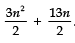. Find the 25th term.
Sol. We know that: an = Sn - Sn-1, where,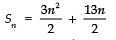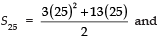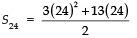Now, a25 = S25 − S24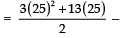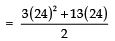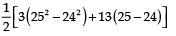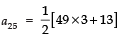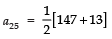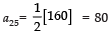Q28. The sum of 4th and 8th terms of an A.P. is 24 and the sum of 6th and 10th terms is 44. Find the first three terms of the A.P.
Sol. Let the first term be ‘a’ and the common difference be ‘d’.
Using T= a + (n - 1) d, we have
T4 = a + 3d, T= a + 5d
T8 = a + 7d and T10 = a + 9d
Since T4 + T8 = 24
∴ a + 3d + a + 7d = 24
⇒ 2a + 10d = 24 ⇒ a + 5d = 12   ...(1)
Also, T6 + T10 = 44
∴  a + 5d + a + 9d = 44
⇒ 2a + 14d = 44 ⇒ a + 7d = 22   ...(2)
Subtracting (2) from (1), we get,
a + 7d - a - 5d = 22 - 12
⇒ 2d = 10 ⇒ d = 5
Now from (1),
a + 5 (5) = 12
⇒ a + 25 = 12 ⇒ a = - 13
∴ First term (T1) = a + 0 = - 13
Second term (T2) = a + d
= - 13 + 5 = - 8
Third term T3 = - a + 2d
= - 13 + 10 = - 3

Q29. In an A.P., the first term is 8, nth term is 33 and sum of first n terms is 123. Find n and d, the common difference.
Sol. Here,
First term T1 = 8 ⇒ a = 8
nth term Tn = 33 = l
∵ Sn = 123 [Given]
∴ Using, Sn = n/2 [a + l], we have
Sn = n/2 [8 + 33]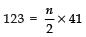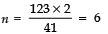Now, T6 = 33
⇒ a + 5d = 33
⇒ 8 + 5d = 33
⇒ 5d = 33 - 8 = 25
⇒ d = 25/5 = 5
Thus, n = 6 and d = 5.

Q30. For what value of n are the nth terms of two A.P.’s 63, 65, 67, ..... and 3, 10, 17, ..... equal?
Sol. For the 1st A.P.
a = 63
d = 65 - 63 = 2
∴ Tn = a + (n - 1) d
⇒ Tn = 63 + (n - 1) × 2
For the 2nd A.P.
a = 3
d = 10 - 3 = 7
∴ Tn = a + (n - 1) d
⇒ Tn = 3 + (n - 1) × 7
∵ [Tn of 1st A.P.] = [Tn of 2nd A.P.]
∴ 63 + (n - 1) × 2 = 3 + (n - 1) × 7
⇒ 63 - 3 + (n - 1) × 2 = (n - 1) 7
⇒ 60 + (n - 1) × 2 - (n - 1) × 7 = 0
⇒ 60 + (n - 1) [2 - 7] = 0
⇒ 60 + (n - 1) × (- 5) = 0
⇒ (n - 1) = -60/-5 = 12
⇒ n = 12 + 1 = 13
Thus, the required value of n is 13.

Q31. If m times the mth term of an A.P. is equal to n times the nth term, find the (m + n)th term of the A.P.
Sol. Let the first term (T1) = a and the common difference be ‘d’.
∴ nth term = a + (n - 1) d
And mth term = a + (m - 1) d
Also,
(m + n)th term = a + (m + n - 1) d   ...(1)
∵ m (mth term) = n (nth term)
∴ m [a + (m - 1) d] = n [a + (n - 1) d]
⇒ ma + m (m - 1) d = na + n (n - 1) d
⇒ ma + (m2 - m) d - na - (n2 - n) d = 0
⇒ ma - na + (m2 - m) d - (n2 - n) d = 0
⇒ a [m - n] + [m2 - m - n2 + n] d = 0
⇒ a [m - n] + [(m2 - n2) - (m - n)] d = 0
⇒ a [m - n] + [(m + n) (m - n) - (m - n)] d = 0
⇒ a [m - n] + (m - n) [m + n - 1] d = 0
Dividing throughout by (m - n), we have:
a + [m + n - 1] d = 0
⇒ a + [(m + n) - 1] d = 0     ...(2)
⇒ (m + n) th term = 0 [From (1) and (2)]

Q32. In an A.P., the first term is 25, nth term is - 17 and sum of first n terms is 60. Find ‘n’ and ‘d’, the common difference.
Sol. Here, the first term a = 25
And the nth term = - 17 = l
Using Tn = a + (n - 1) d, we have:
- 17 = 25 + (n - 1) d
⇒ (n - 1) d = - 17 - 25 = - 42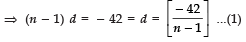Also, Sn = n/2 [a + l]
⇒ 60 = n/2 [25 + (- 17)]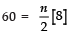⇒ 60 = 4n ⇒ n = 60/4 = 15
From (1), we have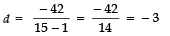Thus, n = 15 and d = - 3

Q33. In an A.P., the first term is 22, nth term is - 11 and sum of first n terms is 66. Find n and d, the common difference.
Sol. We have
1st term (T1) = 22 ⇒ a = 22
Last term (Tn) = - 11 ⇒ l = - 11
Using, Sn = n/2 [a + l], we have:
66 = n/2 [22 + (- 11)]
⇒ 66 × 2 = n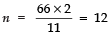Again using
Tn = a + (n - 1) d
We have:
T12 = 22 + (12 - 1) × d
- 11 = 22 + 11d [∵ nth term = - 11]
⇒ 11d = - 22 - 11 = - 33
⇒ d = -33/11 = -3
Thus, n = 12 and d = - 3

Offer running on EduRev: Apply code STAYHOME200 to get INR 200 off on our premium plan EduRev Infinity!

132 docs

,

,

,

,

,

,

,

,

,

,

,

,

,

,

,

,

,

,

,

,

,

;